Question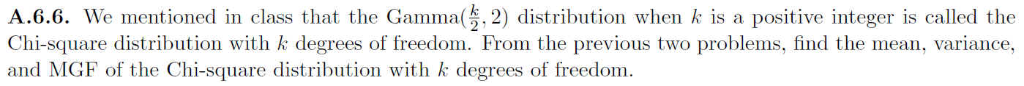The previous two questions mentioned above are included below: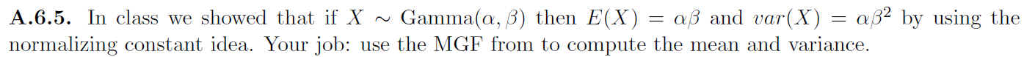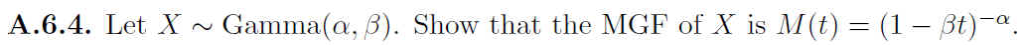A.6.6. We mentioned in class that the Gamma(, 2) distribution when k is a positive integer is called the Chi-square distribution with k degrees of freedom. From the previous two problems, find the mean, variance, and MGF of the Chi-square distribution with k degrees of freedom.
A.6.5. In class we showed that if X ~ Gamma(α, β) then E (X) = aß and uar(X) = αβ2 by using the normalizing constant idea. Your job: use the MGF from to compute the mean and variance.
A.6.4. Let X ~ Gamma(a, 3). Show that the MGF of X is M(t) (1-St)-α.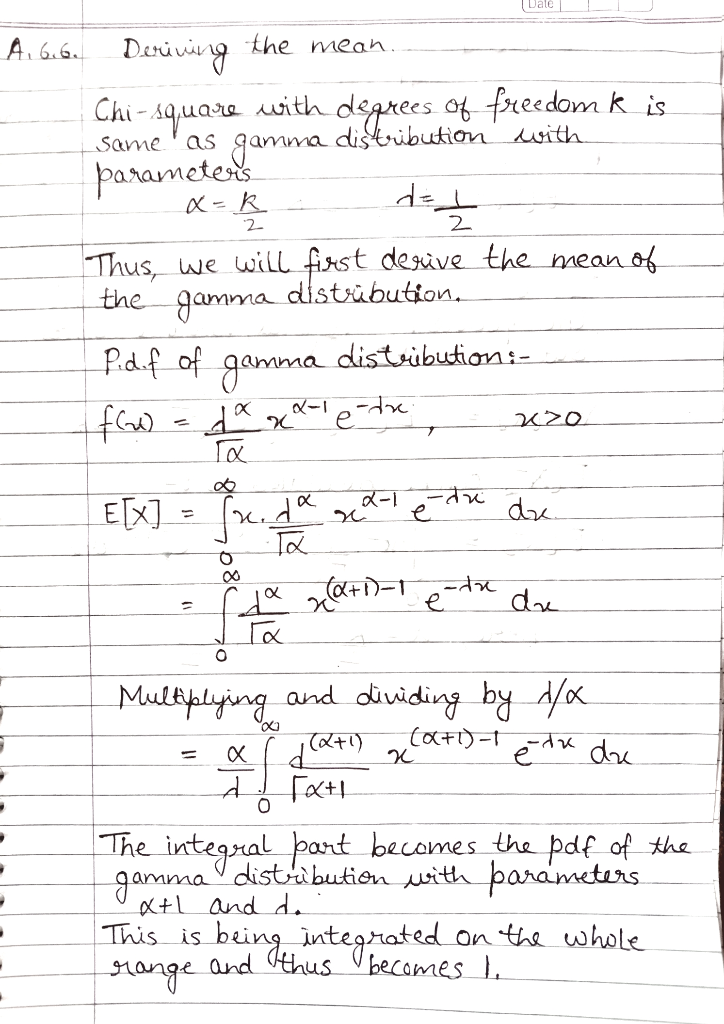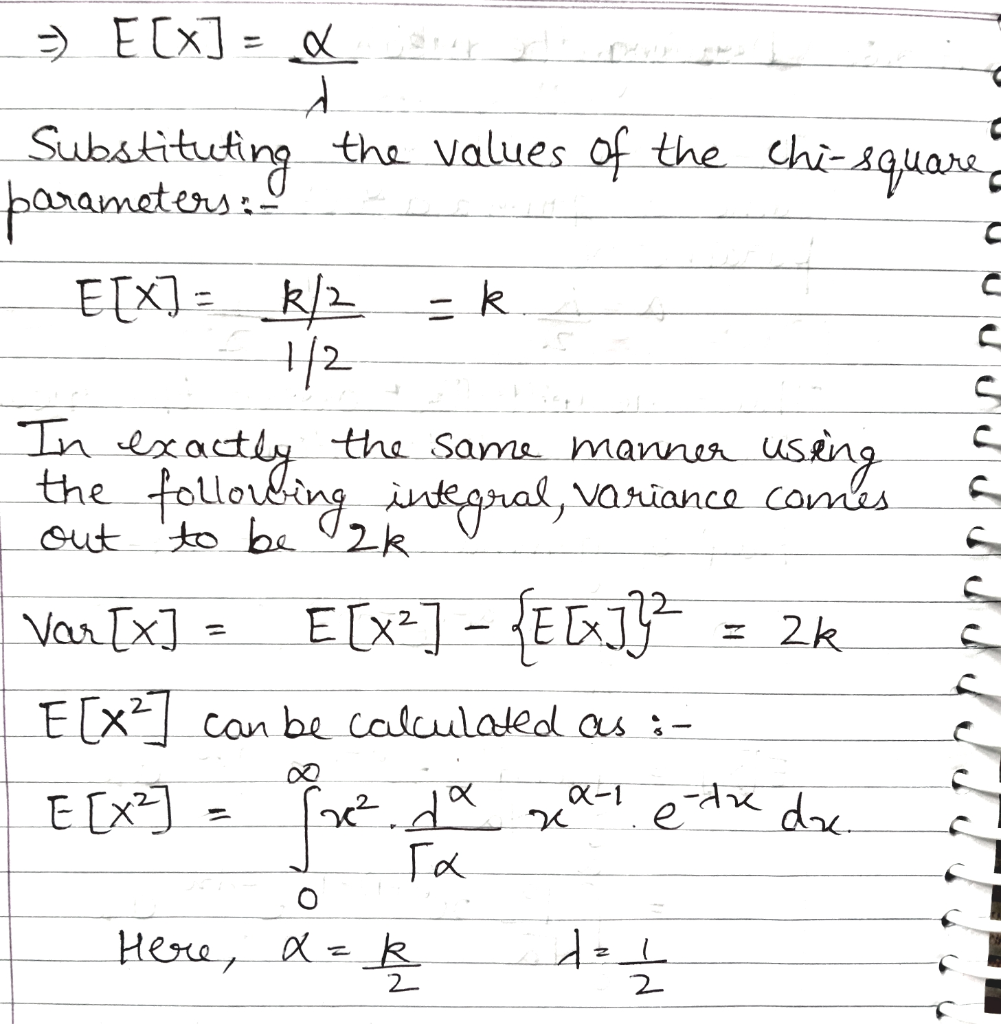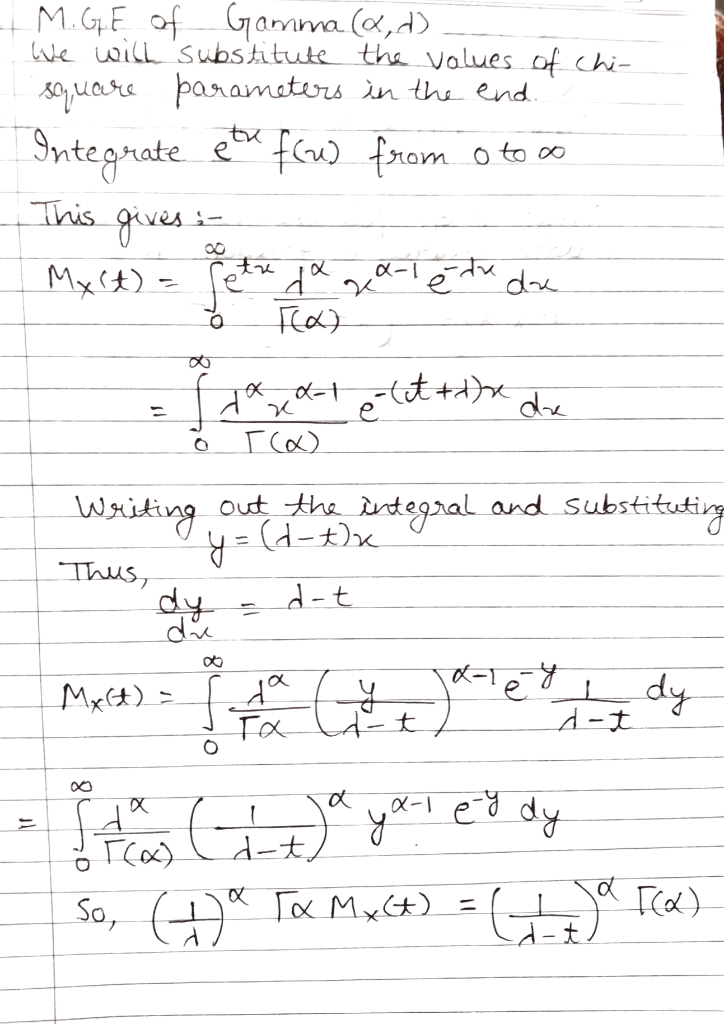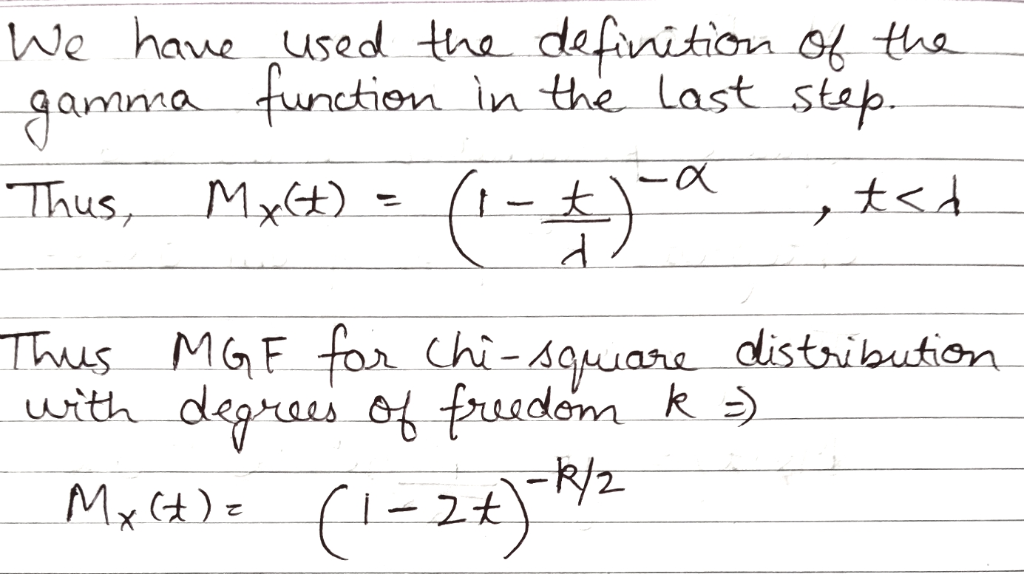#### Earn Coins

Coins can be redeemed for fabulous gifts.

Similar Homework Help Questions
• ### Having troubles with question 2. Please help 2. If X has a Gamma distribution with parameters...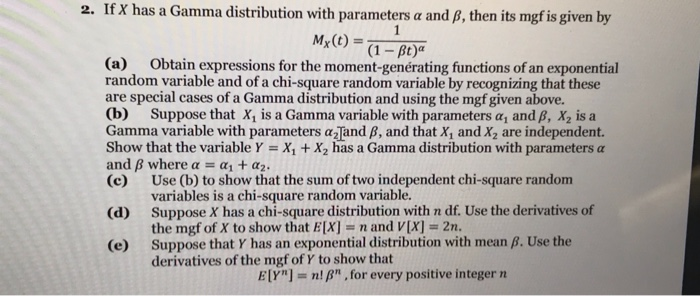Having troubles with question 2. Please help 2. If X has a Gamma distribution with parameters a and B, then its mgf is given by (a) Obtain expressions for the moment-genérating functions of an exponential random variable and of a chi-square random variable by recognizing that these are special cases of a Gamma distribution and using the mgf given above. (b) Suppose that X1 is a Gamma variable with parameters α1 and β, X2 is a Gamma variable with parameters...

• ### 10. (9 pts) Suppose that Yhas a gamma distribution with a -n/2 for some positive integer...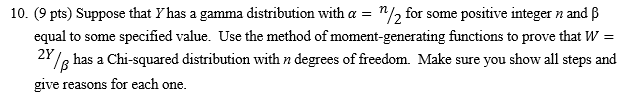10. (9 pts) Suppose that Yhas a gamma distribution with a -n/2 for some positive integer n and β equal to some specified value. Use the method of moment-generating functions to prove that W 2% has a Chi-squared distribution with n degrees of freedom. Make sure you show all steps and give reasons for each one.

• ### Suppose Y1 ~ Gamma(α-5, β-4), Y2 ~ Gamma(α-6, β-4) and Y3 ~ Gamma(α-7, β-4) Furthermore, Yi,...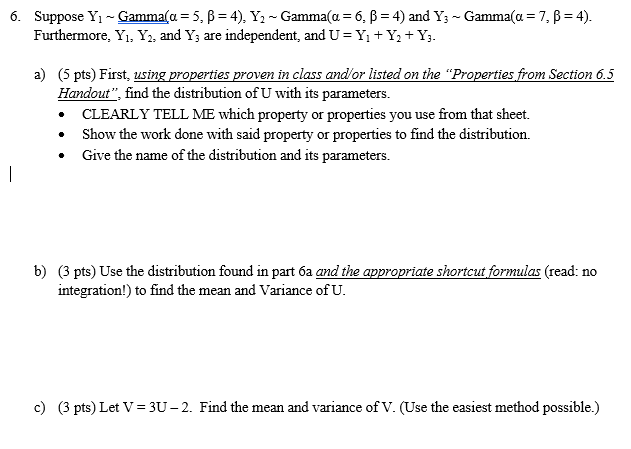Suppose Y1 ~ Gamma(α-5, β-4), Y2 ~ Gamma(α-6, β-4) and Y3 ~ Gamma(α-7, β-4) Furthermore, Yi, Y2, and Yj are independent, and U Y+Y2+ Y 6. a) (5 pts) First, using properties proven in class and/or listed on the "Properties from Section 6.5 Handout", find the distribution of U with its parameters. CLEARLY TELL ME which property or properties you use from that sheet. *Show the work done with said property or properties to find the distribution. . Give the...

• ### LU 22 2. We know that the sample variance follows a chi-square distribution: Sanx?(n-1). (a) (5...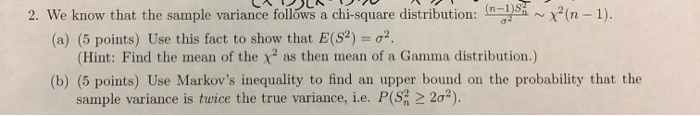LU 22 2. We know that the sample variance follows a chi-square distribution: Sanx?(n-1). (a) (5 points) Use this fact to show that E(S) = 02. (Hint: Find the mean of the x as then mean of a Gamma distribution.) (b) (5 points) Use Markov's inequality to find an upper bound on the probability that the sample variance is twice the true variance, i.e. P(S? > 20%).

• ### 2. The chi-square distribution plays a significant role in performing inference on the as- sociation between...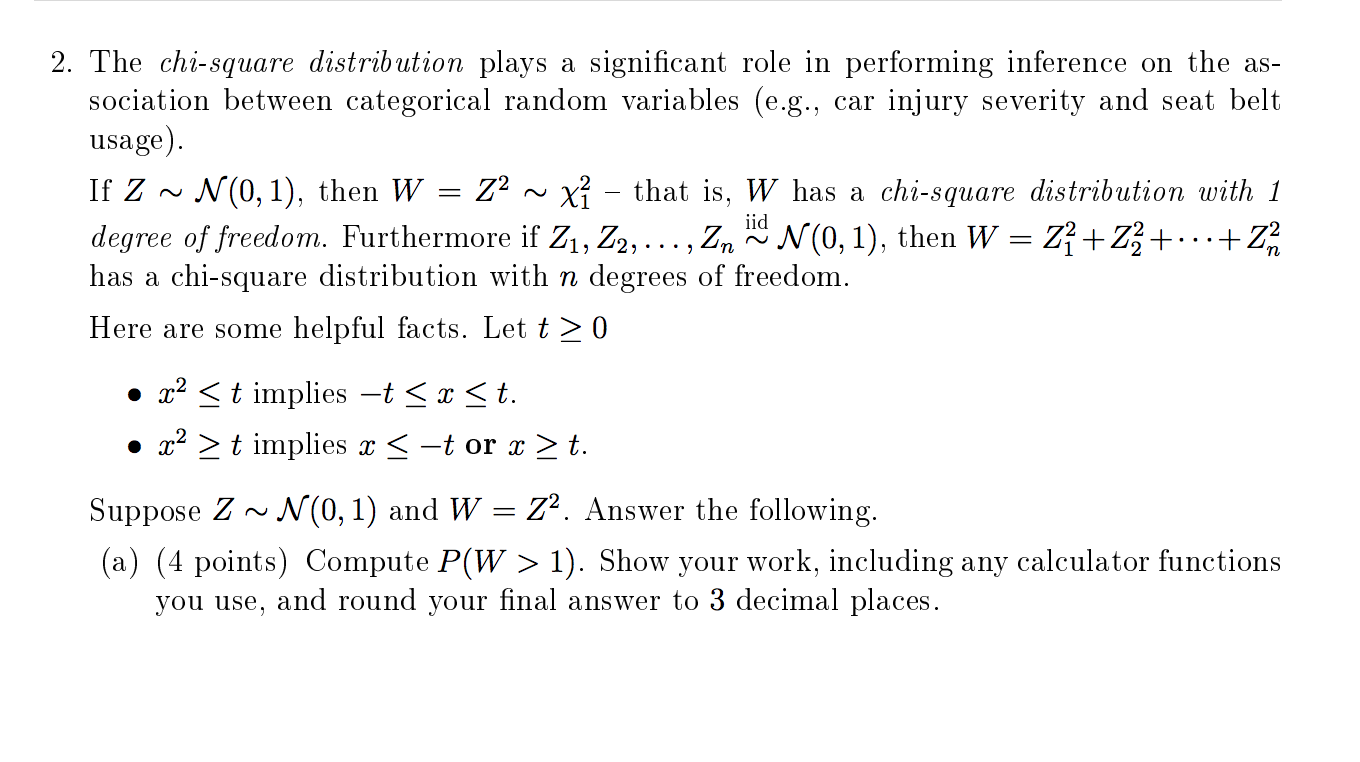2. The chi-square distribution plays a significant role in performing inference on the as- sociation between categorical random variables (e.g., car injury severity and seat belt usage). If Z ~ N(0,1), then W = Z2 ~ xỉ – that is, W has a chi-square distribution with 1 degree of freedom. Furthermore if Z1, Z2, ..., Zn N(0,1), then W = Z+Z2+...+22 has a chi-square distribution with n degrees of freedom. Here are some helpful facts. Let t > 0 •...

• ### 2. This week, we studied the test score Y versus number of hours, X, spent on test preparation, of a student in a French class of 10 students with the collected results shown below Number of hours s...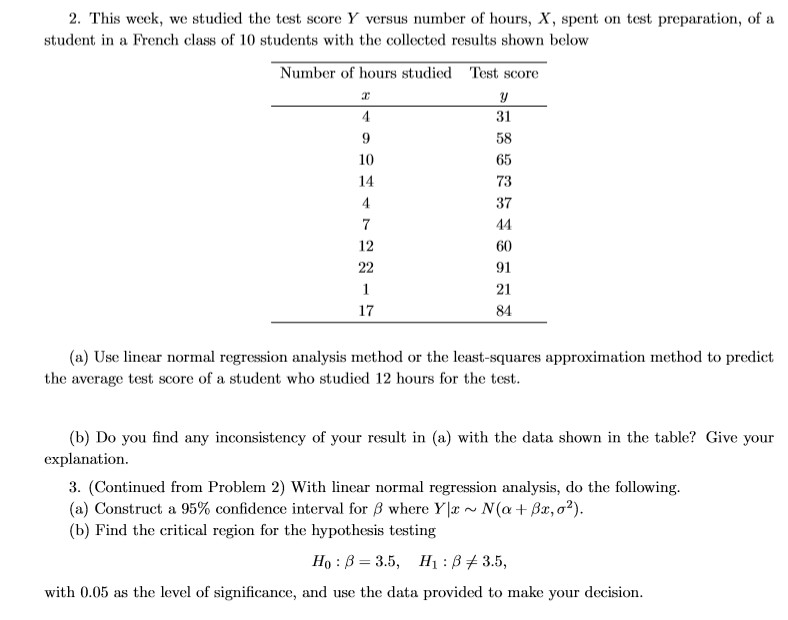2. This week, we studied the test score Y versus number of hours, X, spent on test preparation, of a student in a French class of 10 students with the collected results shown below Number of hours studied Test score 31 10 14 73 37 12 60 91 21 84 17 (a) Use linear normal regression analysis method or the least-squares approximation method to predict the average test score of a student who studied 12 hours for the test (b)...

• ### Practice questions for BIO 340 (Exam 2) I need help with these questions Please. WILL GIVE...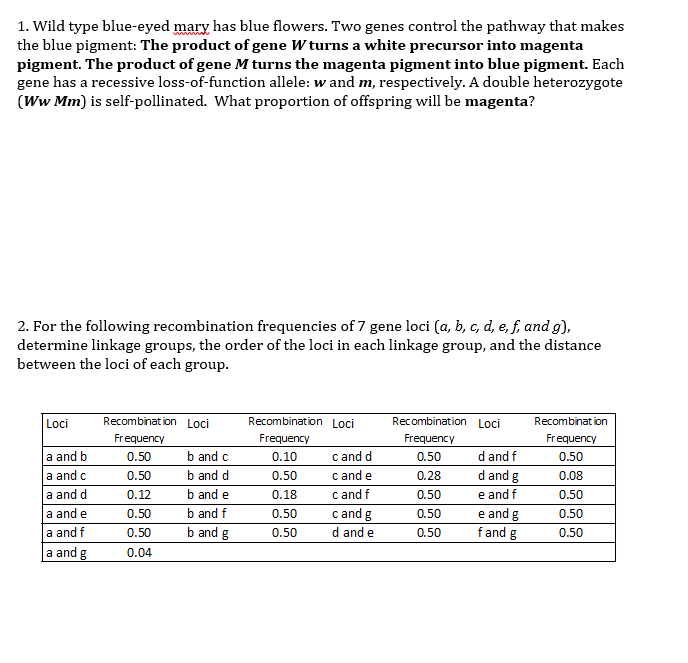Practice questions for BIO 340 (Exam 2) I need help with these questions Please. WILL GIVE GOOD RATING 1. Wild type blue-eyed mary has blue flowers. Two genes control the pathway that makes the blue pigment: The product of gene W turns a white precursor into magenta pigment. The product of gene M turns the magenta pigment into blue pigment. Each gene has a recessive loss-of-function allele: w and m, respectively. A double heterozygote (Ww Mm) is self-pollinated. What proportion...